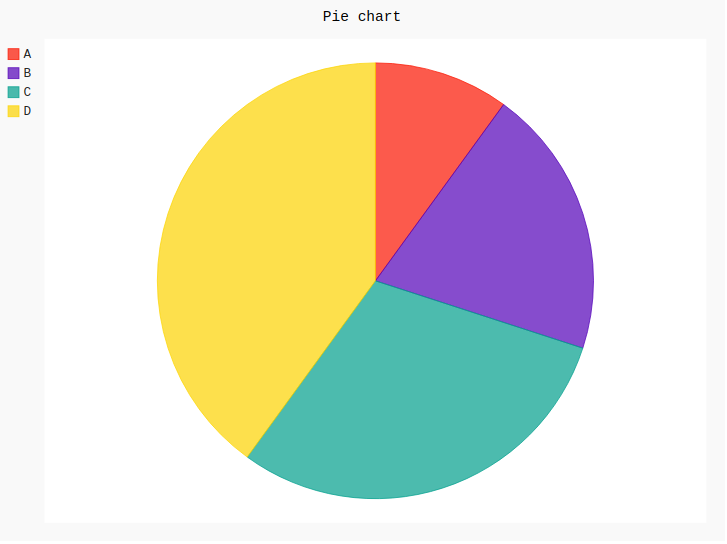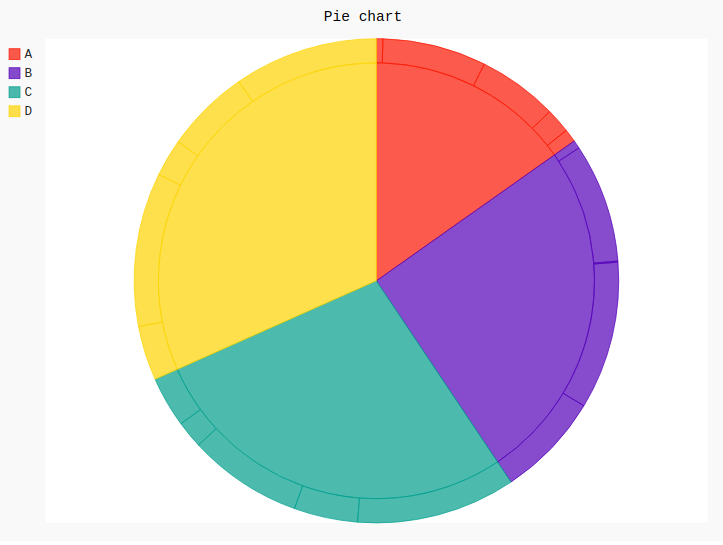# Pie chart in Pygal

Pygal is a Python module that is mainly used to build SVG (Scalar Vector Graphics) graphs and charts. SVG is a vector-based graphics in the XML format that can be edited in any editor. Pygal can create graphs with minimal lines of code that can be easy to understand and write.

## Pie Chart

A pie chart or a circle chart is a chart which is a circular arithmetical graphic, which is divided according to the values to demonstrate numerical proportion and percentage represented by each category is provided next to the corresponding slice of pie. Pie chart is good to represent data in many slices and is very effective while understanding.

A simple pie chart is a chart which represents the data series in one whole pie with a different percentage. Each slice of a pie is proportionally different from the sum of all the data present in a series. It can be created using the `Pie()` method of pygal module.

Syntax:

`pie_chart = pygal.Pie()`

Example 1:

 `# importing pygal ` `import` `pygal ` ` `  `# creating line chart object ` `pie_chart ``=` `pygal.Pie() ` ` `  `# naming the title ` `pie_chart.title ``=` `'Pie chart'` ` `  ` `  `# random data ` `pie_chart.add(``'A'``, ``10``) ` `pie_chart.add(``'B'``, ``20``) ` `pie_chart.add(``'C'``, ``30``) ` `pie_chart.add(``'D'``, ``40``) ` ` `  `pie_chart `

Output:Example 2:

 `# importing pygal ` `import` `pygal ` `import` `numpy ` ` `  ` `  `# creating line chart object ` `pie_chart ``=` `pygal.Pie() ` ` `  `# naming the title ` `pie_chart.title ``=` `'Pie chart'` ` `  ` `  `# random data ` `pie_chart.add(``'A'``, numpy.random.rand(``5``)) ` `pie_chart.add(``'B'``, numpy.random.rand(``5``)) ` `pie_chart.add(``'C'``, numpy.random.rand(``5``)) ` `pie_chart.add(``'D'``, numpy.random.rand(``5``)) ` ` `  `pie_chart `

Output:My Personal Notes arrow_drop_upIf you like GeeksforGeeks and would like to contribute, you can also write an article using contribute.geeksforgeeks.org or mail your article to contribute@geeksforgeeks.org. See your article appearing on the GeeksforGeeks main page and help other Geeks.

Please Improve this article if you find anything incorrect by clicking on the "Improve Article" button below.

Article Tags :

Be the First to upvote.

Please write to us at contribute@geeksforgeeks.org to report any issue with the above content.